Analysis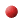The selection condition is equivalent to the choice of the Extreme Value: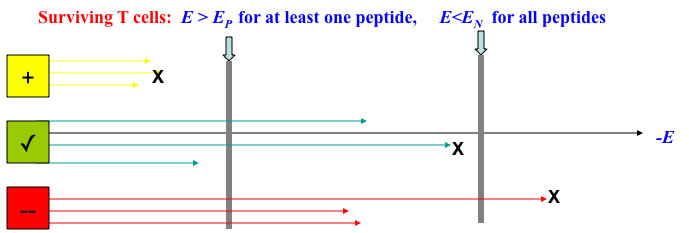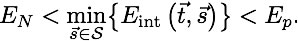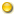Probability distribution of the extremum of M objects: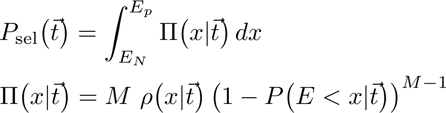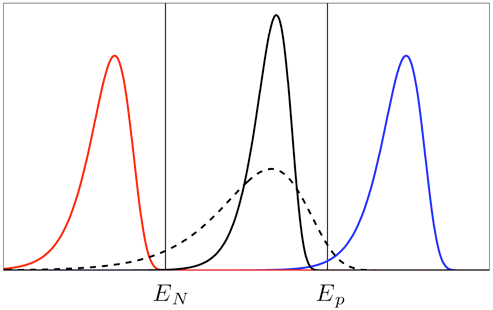Characteristics of the Extreme Value Distribution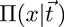:Binding energies of a particular TCR sequencs:Extremal mean value: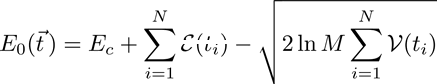~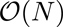,

where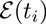and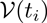are the mean and variance of interactions of the candidateTCR sequence.Extremal standard deviation: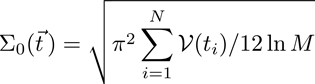~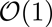Note scaling in the large N limit;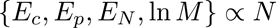(proteome)Due to the shaprpness of the distibution in the large N limit, the seletion condition can be written as: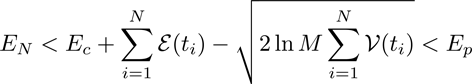[andare the mean and variance of interactions of the candidate TCR sequence.]The above selection condition is reminiscent of the micro-canonical constraints in Statistical Physics.The "energy" involves interactions amongst the N amino-acids in the sequence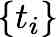The "interaction" depends only on the sum of variances for the individual amino-acidsAs such, in the large N limit, the probability to select a sequence can be written as a product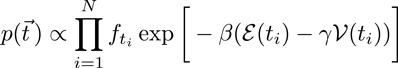,

where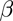andhave to be obtained self-consistently from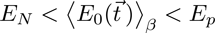and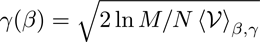.Graphical solution for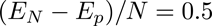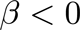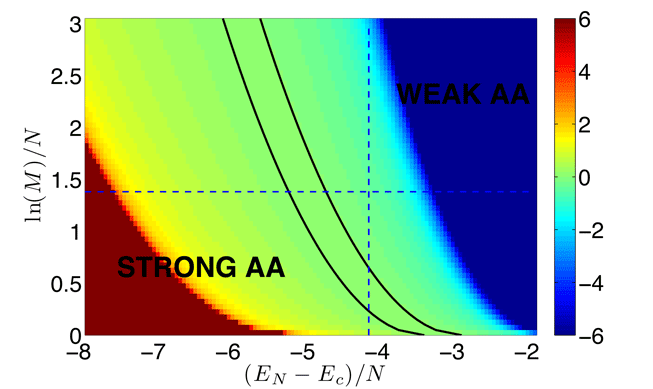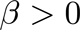Because of the restriction to an energy interval, there is a range of parameters where= 0.How well does this work for finite N? (N=5 and M=10.000)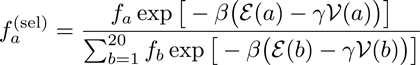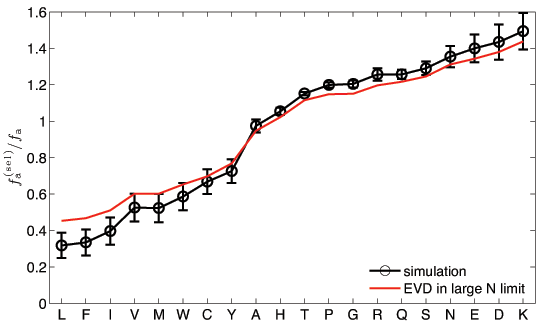A. Kosmrlj, A.K. Chakraborty, M.K., & E. Shakhnovich, PRL 103, 068103 (2009)More elaborate variants of the above model can be studies (Hanrong Chen), such asHow does non-uniform usage of TCR sites, modify selection bias at different sites?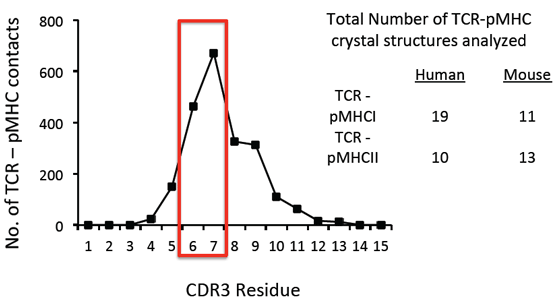Different sites could be in contact with the same amino-acid peptide. What are subsequent correlations between amino-acids at such sites?